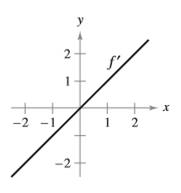Chapter 4.1, Problem 50E

Chapter
Section
Textbook Problem

Sketching a Graph In Exercises 49 and 50, the graph of the derivative of a function is given. Sketch the graphs of two functions that have the given derivative. (There is more than one correct answer.) To print an enlarged copy of the graph, go to MathGraphs.com.To determine

To graph: The two functions that have the same derivative as given below,

Explanation

Given:

The graph of the derivative of function is,

Please Refer the figure in the given question.

Graph:

Consider the given graph,

From the graph, it can be interpreted that,

f(x)=x

Now integrate both sides,

f(x)=x22+C

There are many function associated with the above function. Therefore, two function can be assumed. The first one is,

f(x)=x22

To draw the graph, make the table,

 x −2 −1 0 1 2 f(x) 2 12 0 12

Still sussing out bartleby?

Check out a sample textbook solution.

See a sample solution

The Solution to Your Study Problems

Bartleby provides explanations to thousands of textbook problems written by our experts, many with advanced degrees!

Get Started

Find the derivative of the function. U(y)=(y4+1y2+1)5

Single Variable Calculus: Early Transcendentals, Volume I

Convert the expressions in Exercises 6584 to power form. xx

Finite Mathematics and Applied Calculus (MindTap Course List)

In problems 45-62, perform the indicated operations and simplify. 60.

Mathematical Applications for the Management, Life, and Social Sciences

Define applied research and basic research and identify examples of each.

Research Methods for the Behavioral Sciences (MindTap Course List)